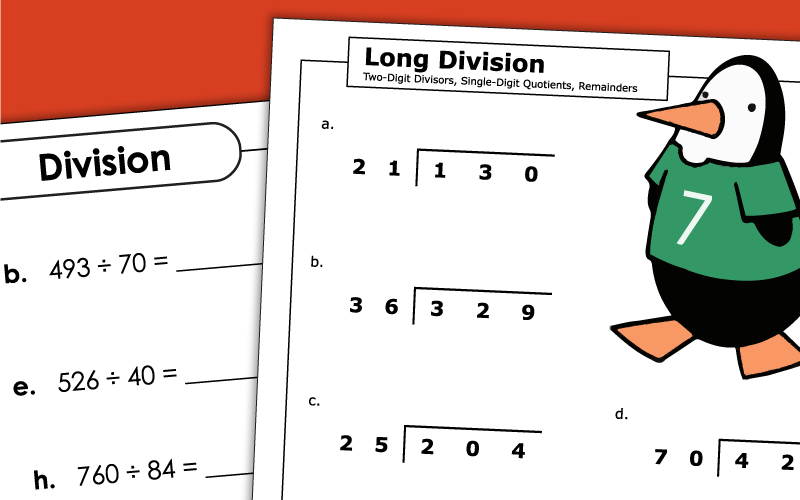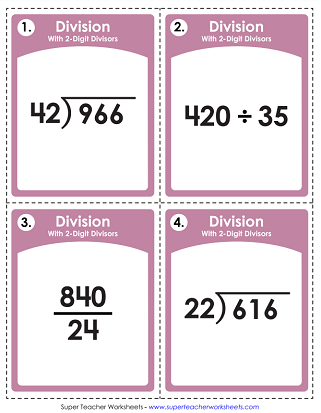# Long Division with 2-Digit Divisors

The printable worksheets on this page have 2-digit divisors. The files below include traditional practice worksheets (with and without remainders), word problems, and graph paper math.## 2-Digit Divisors2 and 3-Digit Dividends

Long division with two-digit divisors; no remainders; Car wash picture and word problem.
Two-digit divisors with remainders; Penguin picture and word problem.
Students rewrite each problem in long division form and solve. Each divisor has 2-digits.
These task cards feature division problems with 2-digit divisors. Problems are listed traditionally, horizontally, and as fractions.
This set has 30 task cards with division facts. Use them for classroom scavenger hunts, exit slips, 1-on-1 tutoring, or on your document camera.
These problems have 2-digit divisors, 3 and 4-digit dividends. All quotients are whole numbers.

## Long DivisionWorksheet Generator

Division Worksheet Generator

Create your own long division worksheets! You choose the number of digits in the dividend and the divisor. Also tell if you would like remainders.

## 2-Digit Divisors4-Digit Dividends

This page has long division problems with 2-digit divisors and 4-digit dividends.
This page has 9 problems for students to complete. Each one has a 2-digit divisor and a 4-digit dividend.
More Long Division

STW has long division worksheets with 2, 3, and 4-digit divisors. We also have sets of printables with both 1 and 2-digit dividends. You will also find activities to review dividing money and decimals.

## Sample Worksheet ImagesMy Account
Site Information